## Thursday, April 28, 2016

### Multi View Stereo 10 (MVS10)

MVS10 builds a dense reconstruction of a 3D scene from a set of pictures (taken with a camera in photo mode or extracted from a video). Its input is a nvm file which comes either from Structure from Motion 10 (SfM10) or VisualSFM : A Visual Structure from Motion System and the images. The nvm file contains the camera poses (location and orientation) as well as a sparse 3D reconstruction of the scene. MVS10 is loosely based upon "Bundled Depth-Map Merging for Multi-View Stereo" by Jianguo Li, Eric Li, Yurong Chen, Lin Xu, Yimin Zhang. MVS10 pairs selected images/cameras, computes the corresponding disparity maps, and merges them as best as possible.

If you go to the 3D Software page and download/extract ugosoft3d-10-x64.rar, you will find a manual for MVS10 called "mvs10_manual.pdf". The manual explains the workflow/pipeline of MVS10 and the parameters in "mvs10_input.txt", the input file needed by MVS10. In order to use MVS10, you need to run Structure from Motion 10 (SfM10) first.

The output of MVS10 is:
- A set of frames named duh_XX.jpg where XX varies from 01 to 11 that can be used to create an animated gif of the reconstructed 3D scene.
- A point cloud in ply format names duh.ply that describes the 3D scene which can be loaded and possibly meshed (I recommend using the Poisson method) in MeshLab.

Here are a few examples:

Here's a tutorial video for MVS10:

The windows executable (guaranteed to be virus free) is available for free via the 3D Software Page.

Source code: MVS10 on github.

## Thursday, April 14, 2016

### Multi View Stereo (MVS) vs Two View Stereo

In this post, we are gonna compare Structure from Motion 10 (SfM10) + Depth Map Automatic Generator 8b (DMAG8b) [Multi View Stereo] against Epipolar Rectification 9b (ER9b) + Depth Map Automatic Generator 5 (DMAG5) [Two View Stereo].

DMAG8b creates a depth map from a series of frames using the nvm file that SfM10 produces. DMAG5 creates a depth map from an image pair rectified by ER9b. It should be noted that DMAG8b is basically the multi view version of DMAG5 (they are using the same parameters).

One would think that the combo SfM10+DMAG8b would create a more accurate depth map because there are more images involved but it is not a given.

Let's first see what kind of depth map the combo SfM10+DMAG8b produces:3D reconstruction by SfM10 using nbr of trials (AC-RANSAC) = 10000, max number of iterations (Bundle Adjustment) = 1000, min separation angle = 1.5, and max reprojection error = 16.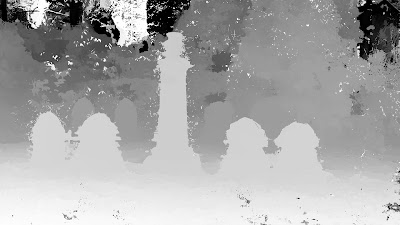Depth map obtained by DMAG8b using near plane = 0, far plane = 0, number of planes = 0, radius = 36, alpha = 0.9, truncation (color)= 30, truncation (gradient) = 10, and epsilon = 4.

I wouldn't worry too much about the wrong depths way back in the background as the actual far plane is probably deeper than the calculated far plane (from the 3D reconstruction).

Now, let's look at the depth map produced by the combo ER9b+DMAG5:

The initial pair chosen by SfM10 is the stereo pair we are gonna use in ER9b. In order to get a depth map (with DMAG5) that shows the foreground as white and the background as black, we need to figure out which one is the left image and which one is the right image. That's pretty easy to tell just by looking at the images.

The min and max disparity that ER9b outputs can be plugged in into DMAG5 as is. If they don't make sense (the range is way too large compared to reality), it definitely means that wrong matches were kept as inliers (the rectified images should be ok though). In that case, one can either try to use ER9 instead of ER9b (don't forget to negate and switch the min and max disparities since ER9 uses a different convention than ER9b for disparities) or use DF2 to manually compute the min and max disparities. In our case, ER9b gives min disparity = -8 and max disparity = 139, which looks mighty fine. Note that the initial pair chosen by SfM10 was the pair of images with index 2 and 3, which means that image 2 is the reference image. For ER9b and DMAG5, the left image is the one with index 3 and the right image the one with index 2. No big deal if you switch those up as the only thing that's gonna happen is that you're gonna get an inverted depth map where black is foreground and white is background.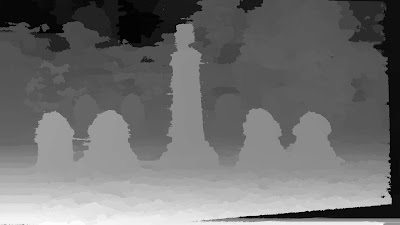Disparity map obtained by DMAG5 using radius = 36, alpha = 0.9, truncation (color)= 30, truncation (gradient) = 10, and epsilon = 4.

This depth map was obtained using the exact same parameters (in DMAG5) as the previous one (DMAG8b). The only difference really is that here (DMAG5) the left and right depth maps are computed in order to detect occlusions which are then erased and filled in using neighboring depths (inpainting).

Which depth map is best? Well, I will let you decide but one can certainly say that the depth map produced by the combo SfM10+DMAG8b is not really better than the depth map produced by the combo ER9b+DMAG5. It should also be noted that, in general, the combo SfM10+DMAG8b is much slower than the combo ER9b+DMAG5.

### Structure from Motion - Tombs and memorials (2)

Here are some 3D scene reconstructions that were obtained with Structure from Motion 10 (SfM10). The frames were extracted from short movies taken by my iphone 4 (resolution=1920x1080). To extract the frames from the videos, I used avidemux, but anything else can be used like VLC.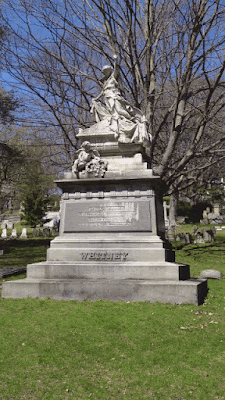Input to SfM10: 7 frames at a resolution of 1920x1080 using a focal length equal to 1920x35/36=1866.Sparse 3D scene reconstructed by SfM10 (number of trials in AC-RANSAC = 1000, max number of iterations in Bundle Adjustment = 1000, min separation angle = 1.5, and max reprojection error = 16).

Hmmm, something went terribly wrong here. Let's hike up the number of trials in AC-RANSAC and see if that's enough to solve the problem.Sparse 3D scene reconstructed by SfM10 (number of trials in AC-RANSAC = 10000, max number of iterations in Bundle Adjustment = 1000, min separation angle = 1.5, and max reprojection error = 16).

Looks like it solved the problem nicely.Input to SfM10: 7 frames at a resolution of 1920x1080 using a focal length equal to 1920x35/36=1866.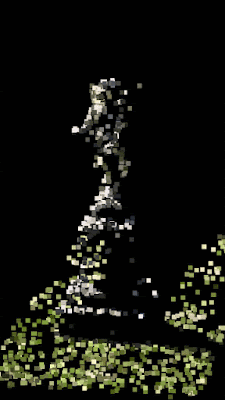Sparse 3D scene reconstructed by SfM10 (number of trials in AC-RANSAC = 1000, max number of iterations in Bundle Adjustment = 1000, min separation angle = 1.5, and max reprojection error = 16).Input to SfM10: 6 frames at a resolution of 1920x1080 using a focal length equal to 1920x35/36=1866.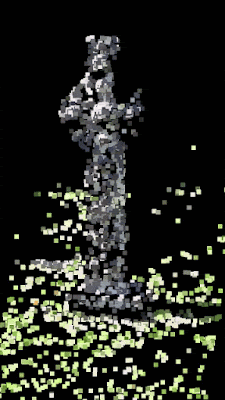Sparse 3D scene reconstructed by SfM10 (number of trials in AC-RANSAC = 1000, max number of iterations in Bundle Adjustment = 1000, min separation angle = 1.5, and max reprojection error = 16).Input to SfM10: 5 frames at a resolution of 1920x1080 using a focal length equal to 1920x35/36=1866.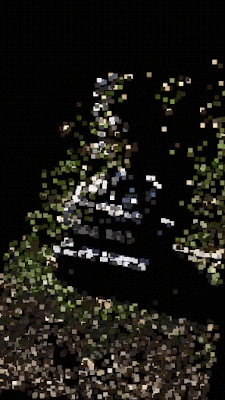Sparse 3D scene reconstructed by SfM10 (number of trials in AC-RANSAC = 1000, max number of iterations in Bundle Adjustment = 1000, min separation angle = 1.5, and max reprojection error = 16).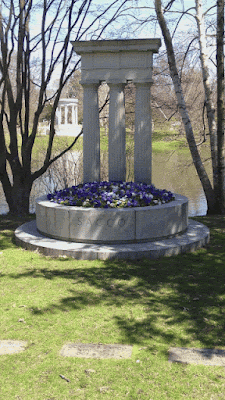Input to SfM10: 8 frames at a resolution of 1920x1080 using a focal length equal to 1920x35/36=1866.Sparse 3D scene reconstructed by SfM10 (number of trials in AC-RANSAC = 1000, max number of iterations in Bundle Adjustment = 1000, min separation angle = 1.5, and max reprojection error = 16).

Recall that the nvm file that SfM10 outputs can be used to generate a dense depth map (Multi View Stereo) with Depth Map Automatic Generator 8 or Depth Map Automatic Generator 8b (DMAG8b.

### Structure from Motion - Tombs and memorials

Here are some 3D scene reconstructions that were obtained with Structure from Motion 10 (SfM10). The frames were extracted from short movies taken by my iphone 4 (resolution=1920x1080). To extract the frames from the videos, I used avidemux, but anything else can be used (VLC for example).Input to SfM10: 5 frames at a resolution of 1920x1080 using a focal length equal to 1920x35/36=1866.Sparse 3D scene reconstructed by SfM10 (number of trials in AC-RANSAC = 1000, max number of iterations in Bundle Adjustment = 1000, min separation angle = 1.5, and max reprojection error = 16).Input to SfM10: 5 frames at a resolution of 1920x1080 using a focal length equal to 1920x35/36=1866.Sparse 3D scene reconstructed by SfM10 (number of trials in AC-RANSAC = 1000, max number of iterations in Bundle Adjustment = 1000, min separation angle = 1.5, and max reprojection error = 16).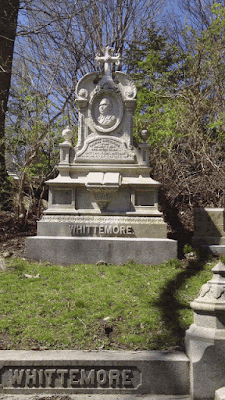Input to SfM10: 5 frames at a resolution of 1920x1080 using a focal length equal to 1920x35/36=1866.Sparse 3D scene reconstructed by SfM10 (number of trials in AC-RANSAC = 1000, max number of iterations in Bundle Adjustment = 1000, min separation angle = 0.5, and max reprojection error = 16).Input to SfM10: 8 frames at a resolution of 1920x1080 using a focal length equal to 1920x35/36=1866.Sparse 3D scene reconstructed by SfM10 (number of trials in AC-RANSAC = 1000, max number of iterations in Bundle Adjustment = 1000, min separation angle = 1.5, and max reprojection error = 16).

Recall that the nvm file that SfM10 outputs can be used to generate a dense depth map (Multi View Stereo) with Depth Map Automatic Generator 8 or Depth Map Automatic Generator 8b (DMAG8b.

## Monday, April 11, 2016

### Structure from Motion - Target aisles

Went to Target and shot a couple of videos using my iphone 4 to test Structure from Motion 10 (SfM10). The iphone 4 captures videos at a resolution of 1920x1080. To extract the frames from the videos, I used avidemux. Two mega pixels per frame is not too bad for SfM10, so I didn't downsize the frames.Input to SfM10: 4 frames at a resolution of 1920x1080 using a focal length equal to 1920x35/36=1866 (number of trials in AC-RANSAC = 1000, max number of iterations in Bundle Adjustment = 1000, and min separation angle = 0.0).Input to SfM10: 5 frames at a resolution of 1920x1080 using a focal length equal to 1920x35/36=1866 (number of trials in AC-RANSAC = 1000, max number of iterations in Bundle Adjustment = 1000, and min separation angle = 1.5).

## Saturday, April 9, 2016

### Structure from Motion 10 (SfM10)

SfM10 (Structure from Motion 10) builds a sparse 3d scene reconstruction given a set of still frames and a focal length (for each frame). Of course, it also computes the camera poses (rotation and translation) for each camera/view.

If you go to the 3D Software page and download/extract ugosoft3d-10-x64.rar, you will find a manual for SfM10 called "sfm10_manual.pdf". The manual explains the workflow/pipeline of SfM10 and the parameters in "sfm10_input.txt", the input file needed by SfM10.

Here are some examples of sparse 3d reconstructions obtained by SfM10:

Here's a tutorial video that explains the process:

The windows executable (guaranteed to be virus free) is available for free via the 3D Software Page.

Source code: SfM10 on github.